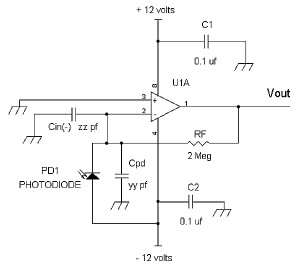# A guide to using FETS for sensor applications, Part 2

In some JFET op amps such as the AD743, the input capacitance is in the order of 18 to 20 pF. In comparison, with an LSK489 dual FET, the input capacitance is in the order of 3 pF, which will be suitable for low noise photodiode applications. In this section we will see why it is important to have low equivalent input noise and low input capacitance in a photodiode preamp.A simple photodiode is shown in Figure 8, which uses an op amp.

In the photodiode amplifier above, when light is shined onto the photodiode, current is generated by the photodiode, PD1. As configured with the cathode of PD1 connected to the (-) input terminal of U1, Vout generates a positive voltage proportional to the amount of light into the photodiode. Also shown in Figure 8 are the equivalent capacitances from the photodiode, Cpd, and (-) input terminal, Cin(-), which are connected in parallel. To minimize Cpd, the photodiode capacitance, the anode of PD1 is connected to the minus 12 volt power supply for maximum reverse bias to lower its junction capacitance. For example, if a BPV10 photodiode is used, Cpd is about 2.7 pF at 12 volts reverse bias. At a lower reverse bias voltage such as 1 volt, Cpd is about 7 pf.

For low noise considerations, these two capacitances, Cpd and Cin(-), should be low as possible. The reason is that the equivalent input noise density voltage, Vnoise_input of the op amp will be amplified in the following manner at Vout, neglecting any noise current from the photodiode:

Vout_noise for a bandwidth of 1 Hz = (Vnoise_input) √(1+ωRFCt)2 + √4kTRF        (1)

Where
ω = 2πfRF = feedback resistor, k = 1.38 x10-23 Joules per degrees Kelvin, T = 298 degrees Kelvin

Ct = Cpd||Cin(-) = total capacitance at the (-) input terminal, and

Ct = Cpd + Cin(-)
√4kTRF = thermal noise voltage of the feedback resistor RF for a bandwidth of 1 Hz.

As we can see from the equation above, the output noise, Vout_noise, goes higher if Ct is increased.

For more Details: A guide to using FETS for sensor applications, Part 2